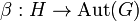# Compatible with trivial action iff image centralizes inner automorphisms

## Statement

Suppose$G$ and$H$ are groups with homomorphisms$\alpha:G \to \operatorname{Aut}(H)$ and$\beta:H \to \operatorname{Aut}(G)$ such that$\alpha$ is the trivial homomorphism, i.e., it sends every element of$G$ to the identity automorphism of$H$.

Then, the following are equivalent:

1.$\alpha$ and$\beta$ form a compatible pair of actions.
2. The image of$\beta$ in$\operatorname{Aut}(G)$ lies inside the centralizer$C_{\operatorname{Aut}(G)}(\operatorname{Inn}(G))$, i.e., it commutes with all the inner automorphisms of$G$.

## Proof

The actions are compatible if and only if the following two conditions hold, where$\cdot$ denotes the action$\alpha,\beta$ or conjugation within a group, as is clear from context:$g_1 \cdot (h \cdot g_2) = (g_1 \cdot h) \cdot (g_1 \cdot g_2) \ \forall \ g_1,g_2 \in G, h \in H$$h_1 \cdot (g \cdot h_2) = (h_1 \cdot g) \cdot (h_1 \cdot h_2) \ \forall g \in G, h_1,h_2 \in H$

Using the triviality of$\alpha$, these conditions are equivalent to:$g_1 \cdot (h \cdot g_2) = h \cdot (g_1 \cdot g_2) \ \forall \ g_1,g_2 \in G, h \in H$$h_1 \cdot h_2 = h_1 \cdot h_2 \ \forall g \in G, h_1,h_2 \in H$

Note that the second condition is vacuously true, so it conveys no information. The first condition is equivalent to saying that the action of$h$ commutes with conjugation by$g_1$, which is equivalent to saying that$\beta(h) \in C_{\operatorname{Aut}(G)}(\operatorname{Inn}(G))$.